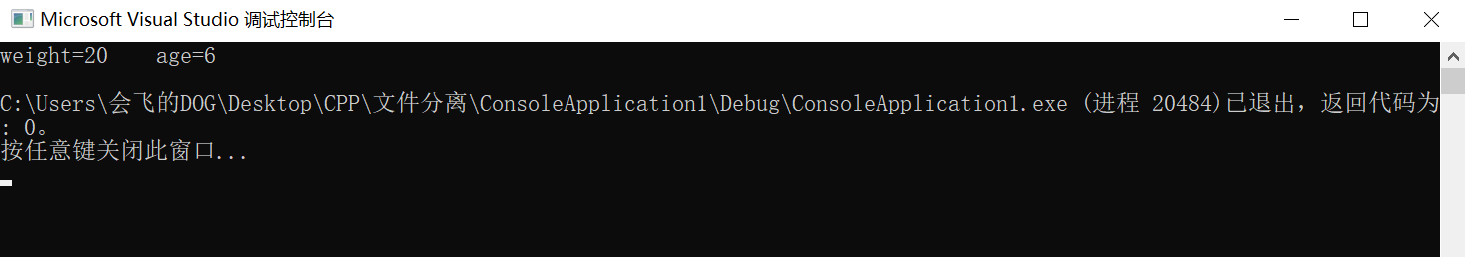# C++第三次作业：友元类

## 友元类

#include "pch.h"
#include <iostream>

using namespace std;

class Dog
{
public:
Dog(int newweight, int newage);
void Show();
friend class B;　　　　　　　　　　　　　　　　　　　　//友元类
private:
int weight;
int age;
};

Dog::Dog(int newweight, int newage)
{
weight = newweight;
age = newage;
}

void Dog::Show()
{
cout << "汪汪"<<endl;
}

class B
{
public:
void print(Dog &a)
{
cout << "weight=" <<  a.weight << "    age=" << a.age <<endl;
}
private:
int number=3;

};
int main()　　　　　　　　　　　　　　　　//主函数测试
{
Dog Tom(20,6);
B Jack;
Jack.print(Tom);
}测试结果表明Dog的友元B是可以去访问Dog的数据的，这样，就实现了数据的共享。

#include "pch.h"
#include <iostream>
using namespace std;

class Dog
{
public:

void Show(B &a);
friend class B;
private:
int weight;
int age;
};

void Dog::Show(B &a)　　　　　　　　　　　　　　//对B类中的数据进行访问
{
cout << a.number<<endl;
}

class B
{
public:
private:
int number=3;
};
int main()
{
Dog Tom;
B Jack;
Tom.Show(B);
}

### 3.友元关系是不能被继承的。

posted @ 2019-09-24 20:51  会飞的DOG  阅读(198)  评论(0编辑  收藏  举报